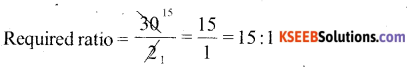# KSEEB Solutions for Class 6 Maths Chapter 12 Ratio and Proportion Ex 12.1

Students can Download Chapter 12 Ratio and Proportion Ex 12.1 Questions and Answers, Notes Pdf, KSEEB Solutions for Class 6 Maths helps you to revise the complete Karnataka State Board Syllabus and score more marks in your examinations.

## Karnataka State Syllabus Class 6 Maths Chapter 12 Ratio and Proportion Ex 12.1

Question 1.
There are 20 girls and 15 boys in a class.
a) What ¡s the ratio of number of girls to the number of boys?
b) What is the ratio of number of girls to the total number of students in the class?
Solution:
Numbers of girls in a class = 20
Numbers of boys in a class = 15
Total numbers of students in a class = 20 + 15 = 35
a) Ratio of number of girls to number of boys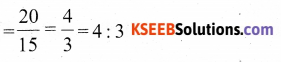b) Ratio of numbers of girls to total number of students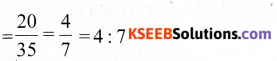Question 2.
Out of 30 students in a class, 6 like football, 12 like cricket and remaining like tennis. Find the ratio of
a) Number of students liking football to numbers of students liking tennis.
b) Numbers of students liking cricket to total number of students.Solution:
Number of students Who like football = 6
number of students Who like cricket = 12
Number of students who like tennis = 30 – 6 – 12 = 12
a) Ratio of the number of students liking football to the number of students liking tennis =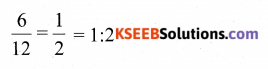b) Ratio of the number of students liking cricket to the total number of students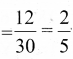= 2 : 5

Question 3.
See that figure and find the ratio of
a) Numbers of triangles to the number of circles inside the rectangle
b) Number of squares to all the figures inside the rectangle
c) Number of circles to all the figures inside the rectangle.Solution:
Number of triangle in a rectangles = 3
Number of circles in a rectangles = 2
Numbers of squares in a rectangles = 2
Total number of figures in a rectangles = 7
a) Ratio of the number of triangles to the number of circles = $$\frac{3}{2}$$
b) Ratio of the number of squares to all the figures in rectangle = $$\frac{2}{7}$$
c) Ratio of the number of circles to tall the figures in the rectangle = $$\frac{2}{3}$$

Question 4.
Distances travelled by Hamid and Akhtar in an hour are 9 km and 12 km. Find the ratio of speed of Hamid to the speed of Akhsta.
Solution:
The distance travelled in an hour by a certain object is caused the speed of the object. Distance travelled by Hamid in one Hour = 9 km / hr
& Distance travelled by Akhtar in one hour = 12 km / hr
Ratio of speed of Hamid to the speed of Akhtar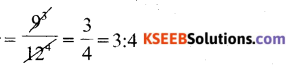Question 5.
Fill in the following Blanks :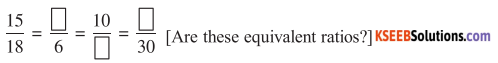Solution: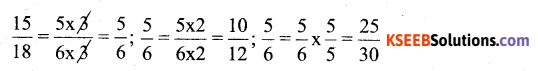Therefore, 5,12, 25 be the answer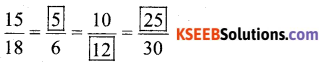True (Yes) all these are equivalent ratios

Question 6.
Find the ratio of the following:
a) 81 to 108
b) 98 to 63
c)33 km to 121 km
d) 30 minutes to 45minutes
Solution: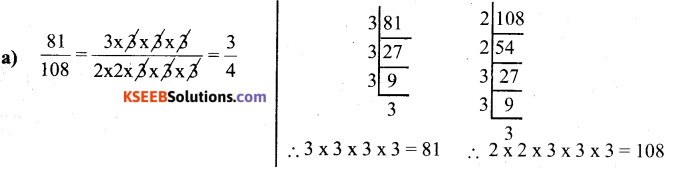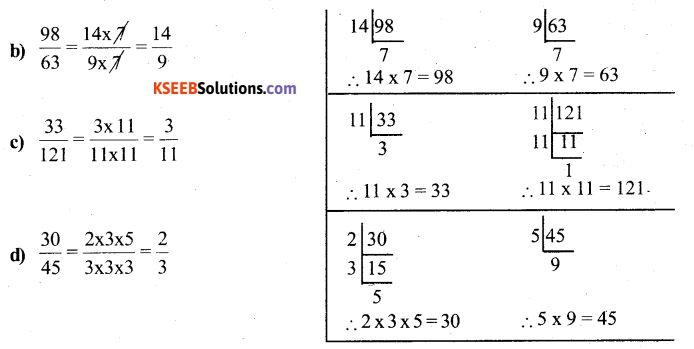Question 7.
Find the ratio of the following
a) 30 minutes to 1.5 hours
b) 40 cm to 1.5 m
c) 55 paise to Rs 1
d) 500 mi to litres
Solution:
a) 1 hour = 60 min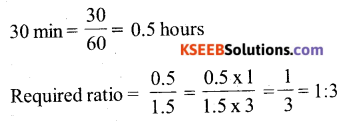b) 40 cm to 1.5 m
1.5 m = 100 cm
1.5 m = 150 cm
Requires Ratio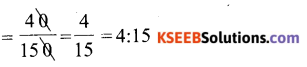c) 55 paise to Rs 1
Rs 1 = 100 paise
Requires Ratio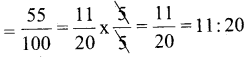d) 500 ml to 2l
1l= 1000 ml
2l = 2 × 1000 ml = 2000 ml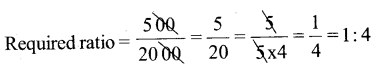Question 8.
In a year, Seema earns Rs 1,50,000 and saves Rs 50,000. Find the ratio of
a) Money that Seema earns to the money she saves.
b) Money that she saves to the money she spends.
Solution:
Money earned by seema = Rs 1,50,000
Money Saved by Seemed = Rs 50,000
Money spent = Rs 1,50,000 – Rs 50,000 = Rs 1,00,000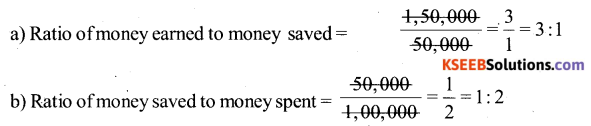Question 9.
There are 102 teachers in a school of 3300 Students, Find the ratio of the number of teachers to the number of students.
Solution: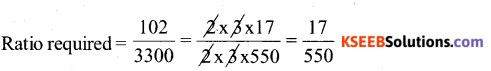Question 10.
In a college, out of 4320 Students, 2300 are girls. Find the ratio of
a) Number of girls to the total number of students.
b) Number of boys to the number of girls.
c) Number of boys to the total number of students
Solution:
Total number of students = 4320
Number of girls in a college = 2300
Number of boys in a college = 4320 – 2300 = 2020
a) Required ratio n for number of girls to the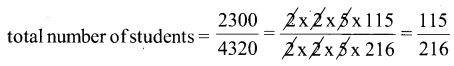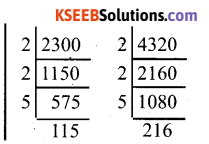b) Required ratio for number of boys to the number of girls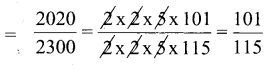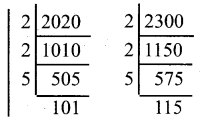c) Required ratio for number of boys to the total number of students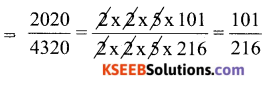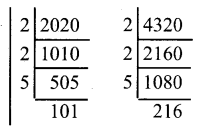Question 11.
Out of 1800 students in a school 750 opted basket ball, 80 opted cricket and remaining opted table tennis. If a student can opt only one game, find the ratio of
a) Number of students who opted basket ball to the number of students Who opted table tennis.
b) Number of students Who opted cricket to the number of students opting basketball
c) Number of students Who opted basket ball to the total number of students.
Solution:
Total number of students = 1800
Number of students opted basketball = 750
Number of students opted cricket = 800
Number of students opted table tennis = 1800 – 750 – 800 = 250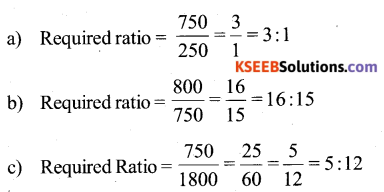Question 12.
Cost of dozen pens is Rs 180 and cost of 8 ball pens is Rs 56. Find the ratio of the cost of pen to the cost of a ball pen.
Solution:
Cost of dozen pens = Rs 180
Cost of 1 per = $$\frac{180}{12}$$ = Rs 15
Cost of 8 ball pens = Rs 56
Cost of ball pens=$$\frac{56}{8}$$ = Rs 7
Required ratio of the cost of a pen to the cost of a ball pen = $$\frac{15}{7}$$ = 15 : 7

Question 13.
Consider the statement: Ratio of breadth and length of a hall is 2 : 5. Complete the following table that shows some possible breadths and lengths of the hall.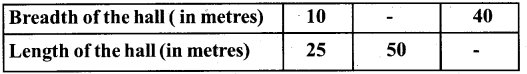Solution:
i) Length = 50 m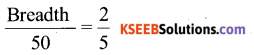5 × Breadth = 50 × 2 (by cross multiplication )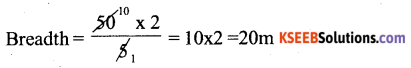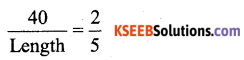2 × Length = 5 × 40 (By cross – Multiplication)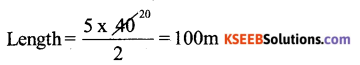Length = 100 m.

Question 14.
Divided 20 pens between sheela and sangeeta in the ratio of 3 : 2.
solution:
Terms of 3 : 2 are 3 and 2
Sum of these two terms = 3 + 2 = 5
Sheela will get $$\frac{3}{5}$$ of total pens and sangeeta will get $$\frac{2}{5}$$ of total pens.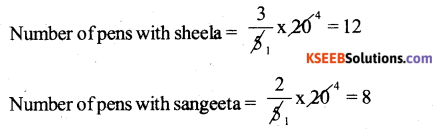Question 15.
Mother wants to divide Rs 36 between her daughters shreya and bhoomika in the ratio of their ages. If age of shreya is 15 years and age of bhoomika is 12 years, find how much shreya and bhoomika will get.
Solution: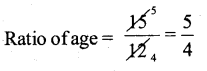Therefore, mother wants to divide Rs 36 in a ratio of 5 : 4 sum of these terms = 5 + 4 = 9
Shreya will get $$\frac{5}{9}$$ of the total money and bhoomika will get $$\frac{4}{9}$$ of it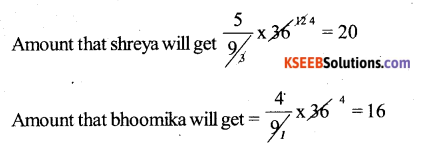Therefore, Shreya and bhoomika will get Rs 20 and Rs 16 respectively.Question 16.
Present age of father is 42 years and that of his son is 14 years. Find the ratio of
a) Present age of father to the present age of son
b) Age of the father to the age of son, When son was 12 years old.
c) Age of father after 10 years to the age of son after 10 years
d) Age of father to the age of son when father was 30 years old
Solution:
a) Present age of father = 42 years
Present age of son = 14 years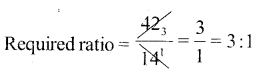b) Two years ago, the age of the son was 12 years and the age of the father was 42 – 2 = 40 years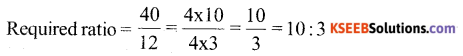c) After 10 years, the age of the father and son will be 52 years and 24 years respectively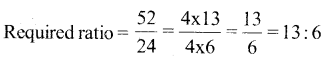d) 12 years ago, the father was 30 years old, At that time age of son = 14 – 12 = 2 years